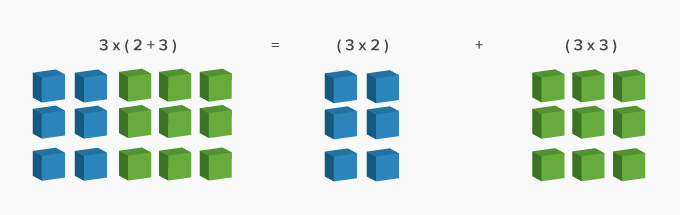# Distributive Property - Definition with Examples

The Complete K-5 Math Learning Program Built for Your Child

• 30 Million Kids

Loved by kids and parent worldwide

• 50,000 Schools

Trusted by teachers across schools

• Comprehensive Curriculum

Aligned to Common Core

## What is Distributive Property?

To “distribute” means to divide something or give a share or part of something.

According to the distributive property, multiplying the sum of two or more addends by a number will give the same result as multiplying each addend individually by the number and then adding the products together.Here’s an example of how the result does not change when solved normally and when solved using the distributive property.

 ( 5 + 7 + 3 ) x 4 = 15 x 4 = 60 ( 5 + 7 + 3 ) x 4 = 5 x 4 + 7 x 4 + 3 x 4 = 60

The distributive property helps in making difficult problems simpler. You can use the distributive property of multiplication to rewrite expression by distributing or breaking down a factor as a sum or difference of two numbers.

Here, for instance, calculating 8 × 27 can made easier by breaking down 27 as 20 + 7 or 30 − 3.

 The distributive property of multiplication over addition: The distributive property of multiplication over subtraction: 8 × ( 20 + 7 ) = 8 × 20 + 8 × 7 = 160 + 56 = 216 8 × ( 30 − 3 ) = 8 × 30 − 8 × 3 = 240 − 24 = 216

 Fun Facts Even though division is the inverse of multiplication, the distributive law only holds true in case of division, when the dividend is distributed or broken down. For instance, using the distributive law for 132  6, 132 can be broken down as 60  60 + 12, thus making division easier. However, 132  (4  2) will give you the wrong result.

###Let's sing!

To multiply big numbers, break one apart,
Multiply with the addends, right at the start,
Now, add the products, part by part.
The answer is right! You’re so smart!

###Let's do it!

Instead of handing out comparison worksheets to your children, you can ask your child to use the distributive property to compute daily life calculations.

For instance, take your child to a stationery store. Ask him to pick out 4 pens and 4 packets of crayons. Ask him to compute the bill amount using the distributive property of multiplication.###Related math vocabulary

Won Numerous Awards & Honors